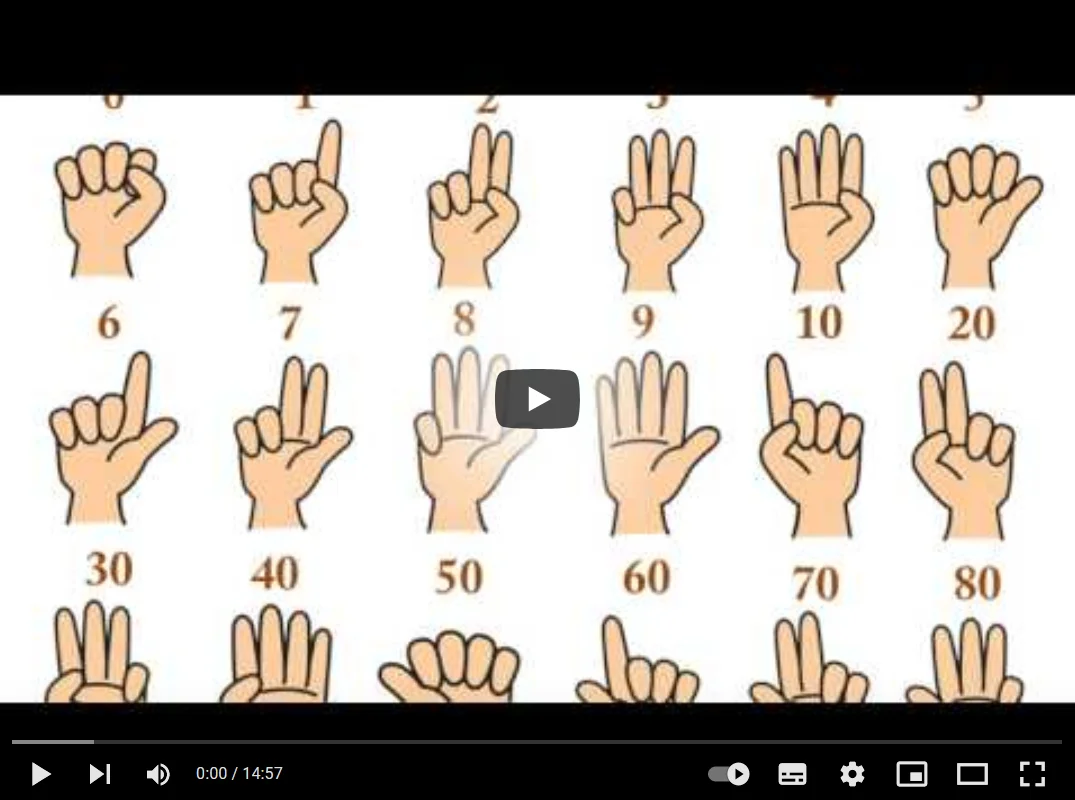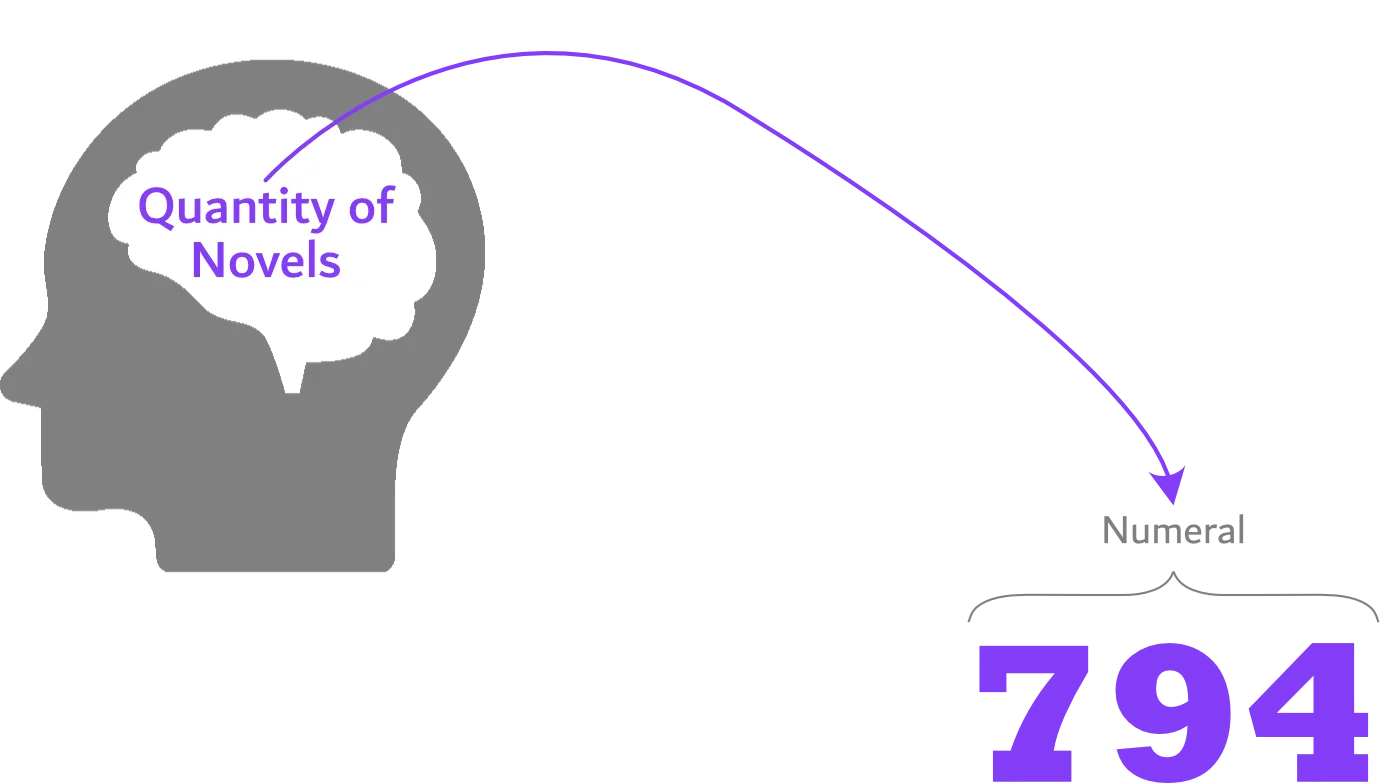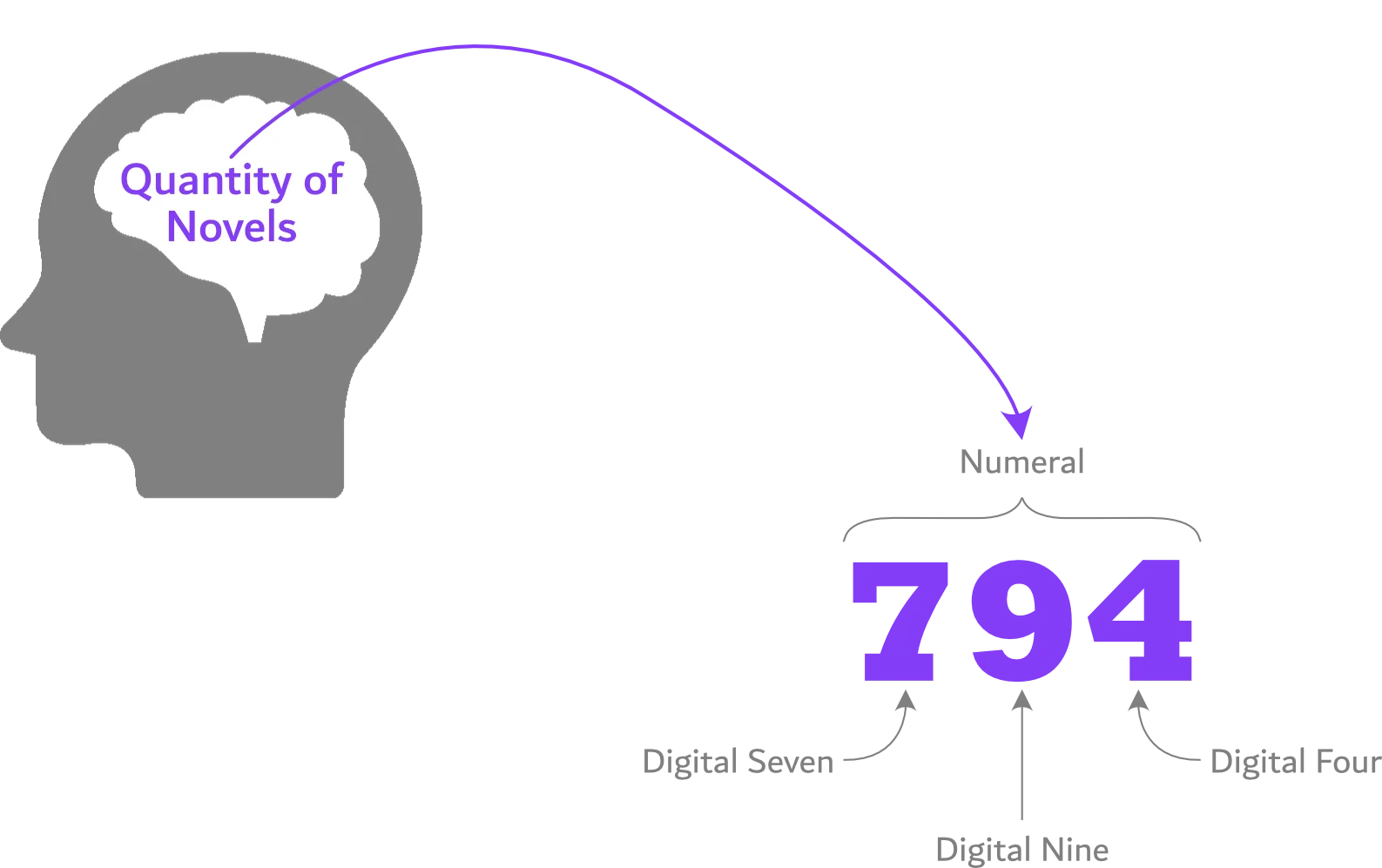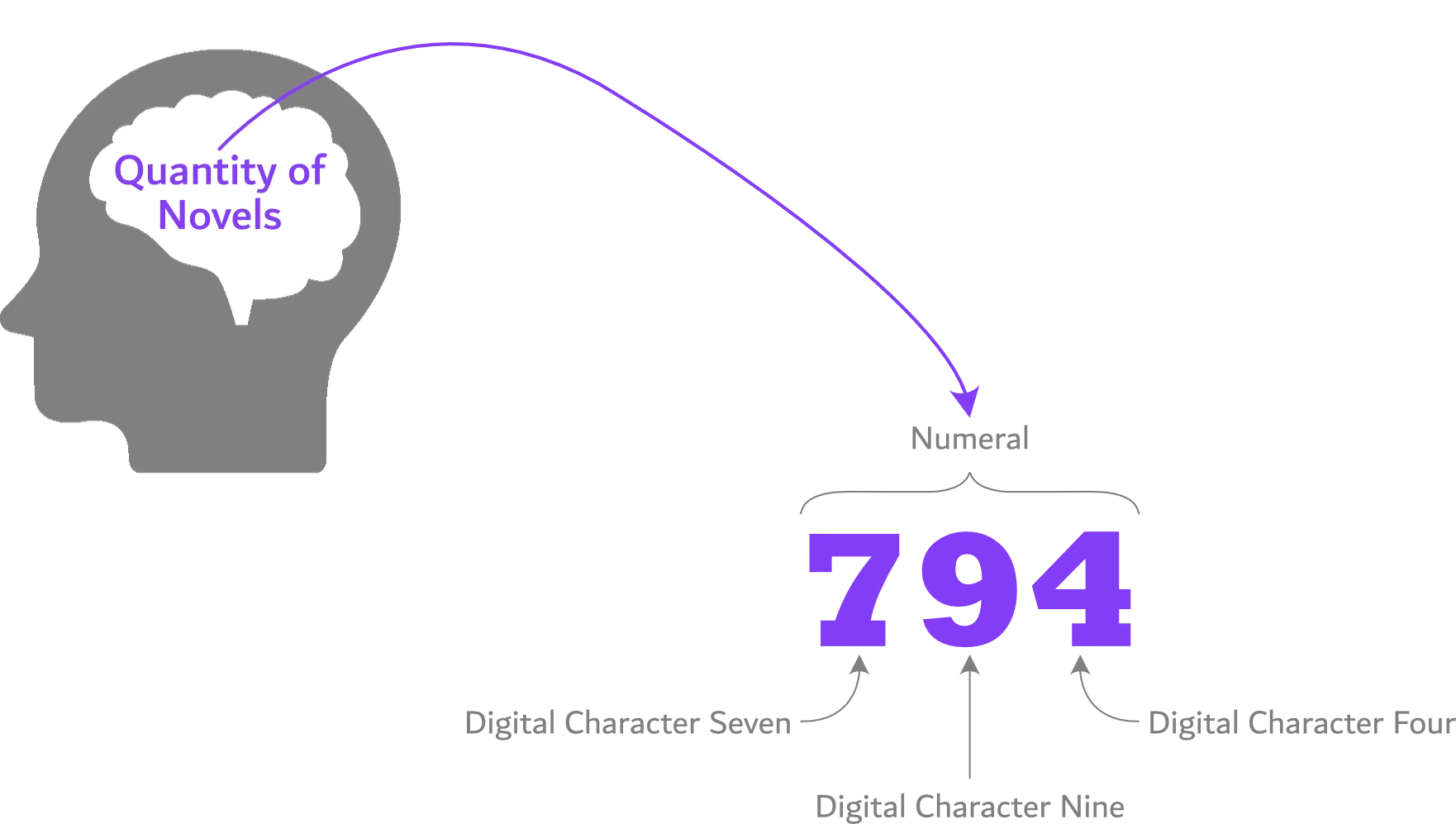# Number vs Numeral vs Digit vs Character

What is the difference between a number, numeral, digit, and character? Let's find out.

## Number​

A number is an idea of quantity.

For instance, suppose you think of the quantity of novels you've read. In such a case, your thought (of the quantity of novels) is a number.There are different ways to translate a number (your thought of quantity) into its physical form.

For instance, you can relate the quantity of novels with your fingers, pieces of bones, tapping the table, symbols, numerals, and so on.## Numeral​

A numeral is the written form of a number.

In other words, suppose you express a number as a writable character. In that case, the character you used to represent the number is the numeral.For instance, the following are numerals used to express the number seven:

note

Amongst the diverse ways of expressing numbers, the Hindu-Arabic numeral is the most common—as it allows multiple ways to do arithmetic quickly and compactly. For instance, the Hindu-Arabic numeral system allows mathematical calculations in binary (base 2), octal (base 8), decimal (base 10), and hexadecimal (base 16) numeral systems.

## Digit​

A digit is each individual character of a numeral—for instance, 794 consists of the digits 7, 9, and 4.## Character​

A character is a writable (or printable) mark.

Some examples of characters are as follows:

• Alphabetical letters (For instance, a, g, and z)
• Digits (For example, 0, 5, and 9)
• Punctuations (For instance, semicolon (;), question mark (?), and colon (:))In the image above, digits 7, 9, and 4 are digital characters.

note
• "185" contains three-character digits: 1, 8, and 5.
• "TEN" contains three-character letters: T, E, and N.
• "=%&@" contains four-character symbols: =, %, &, and @.
• "&61#;" contains five characters (three symbols and two digits): an ampersand sign, digit six, digit one, octothorpe sign, and semicolon sign.

## Overview​

• A number is the abstract form of a quantity.
• A numeral is the written form of a number.
• Digits are the individual characters of a numeral.
• A character is a written (or printed) mark.